• 针对基于单条元路径的异质网络表征缺失异质信息网络中结构信息及其它元路径语义信息的问题，本文提出了基于融合元路径权重的异质网络表征学习方法.该方法对异质信息网络中元路径集合进行权重学习，进而对基于不同元...
•研究论文
• 异质网络将复杂系统中的信息抽象成不同类型的节点和链接关系，不同于同质网络，基于异质网络的社区发现能够挖掘出更加精确的社区结构。异质网络的社区发现通过对异质网络中的多维结构、多模信息、语义信息、链接关系...社区发现 网络结构
• 针对这个问题，提出了一种基于联合矩阵分解的动态异质网络社区发现方法。首先计算动态异质网络中各个快照图的拓扑相似度矩阵和多关系相似度矩阵；其次利用时序联合非负矩阵分解方法，约束各个时刻快照图的社区划分；...动态网络 社区发现 非负矩阵分解
• 这里定义的同质异质网络是指行骗者更可能连接着其他行骗者。合法人更可能连接其他合法人。 令l为网络中合法结点的比例，f为网络中欺诈结点的比例，2lf就是一条边连接两个不同标签的结点的期望可能性，这些边叫做...
当标签x的结点更大程度上连接其他标签x的结点的时候，这个网络是同质的。非同质的网络是异质的。这里定义的同质异质网络是指行骗者更可能连接着其他行骗者。合法人更可能连接其他合法人。令l为网络中合法结点的比例，f为网络中欺诈结点的比例，2lf就是一条边连接两个不同标签的结点的期望可能性，这些边叫做cross-labeled edges.如果已知的cross-labeled edges的比例r小于期望的2lf，这个网络就是同质的。其他衡量同质性的因素还包括dyadicity和heterophilicity.结点分享一个共同的特性比性质随机分散在网络中的可能性更大。这就是dyadicity的影响。对于标签仅仅取两个值的网络，1（Fraud）0（Legitimate），n1(n0)是fraudulent(legitimate)的节点数，N=n0+n1.定义三种dyads:(1-1),(1-0)和(0-0),表示两个终端结点的联系标签（Fraud-Fraud），（Fraud-legitimate）,（Legitamate-legitimate）,每种的数量是m11,m10,m00，M=m11+m10+m00.如果结点随机连接其他的结点而无关于标签，那么m11和m10的期望值相同.m11 = （n1 2）p =n1(n1-1)p/2    m10 = （n1 1）（n0 1）p=n1（N-n1）pp = 2M/（N（N-1））是关联度，代表两个结点连接的可能性。如果p=1，网络中所有结点都相互连接.Dyadicity and heterophilicity然后被这样定义：D=m11/m11, H=m10/m10, 如果D>1则网络是Dyadicity的，表示fraudulent的结点相比随机连接彼此连接更紧密。如果H<1，一个网络是heterophobic的（与heterophilic相反），意味着fraud结点与legitimate结点有更少的连接相比随机的情况。如果一个网络是dyadic and heterophobic,他表现同质性。一个网络是anti-dyadic and heterophilic，是相反的同质性。
展开全文• 本文首先通过分析现有的相似性度量算法的优势与不足，提出了一...该算法可以度量异质信息网络中任意结点对之间的相似度，同时度量具有对称性。通过与其它度量算法在真实数据集上的实验结果的比较，验证了算法的有效性。
• 为了分析DBLP数据中的多种类型的实体信息，挖掘其中特定的知识，首先根据异质网络Graph OLAP（图联机分析处理）模型，建立相应的数据仓库模型；然后依据实体维的概念，构建多维异质图立方模型；最后针对 Graph OLAP...
• 异质网络表征学习的研究进展》基础信息挑战 基础信息 包含不同类型节点和边的为异质信息网络。 元路径是定义在网络模式上的链接两类对象的一条路径 挑战 异质信息网络的复杂性也为网络表征学习提出了新的挑战...
文章链接: link.
基础信息
包含不同类型节点和边的为异质信息网络。 元路径是定义在网络模式上的链接两类对象的一条路径
挑战
异质信息网络的复杂性也为网络表征学习提出了新的挑战：
节点和边的异质性带来的挑战。不同类型的节点和边代表不同的对象，因此，在异质网络的表征学习中需要考虑将不同类型的对象映射到不同的空间中；异质网络中的各类信息蕴含着丰富的语义，它从多个维度来刻画节点的意义，因此，如何有效地抽取和利用异质网络的多维度信息，并有效地融合这些信息以便全面地学习节点的表示是一个巨大的挑战
展开全文• 分析了无线链路的特点及其对TCP性能的影响，讨论和评价了现存的各种异质网络数据传输控制机制，最后指出该领域的研究动向及进一步的工作。拥塞控制
• 网络游戏-对异质网络中对象进行排序的方法.zip
• 网络游戏-在异质网络中操作的无线发射接收单元与系统.zip
• ， 那么是异质(heterogeneous)网络；如果T=1，那么是同质（homogeneous）网络。 2. 事件(event) ： Q i Q_i 可以表示为 < V i , w i > , w_i> ，其中 w i w_i 是事件 Q i Q_i 的权重； V i = { V t i } T t = ...
hyper graph是一种广义上的图，它的边可以连接任意数量的定点。[维基百科](https://zh.wikipedia.org/wiki/%E8%B6%85%E5%9B%BE)。超图是一个集合组

H=<X,E>
$H=$, X是一个有限集合，该集合的元素称为节点或顶点；E是X的非空子集的集合，成为超边（hyper edge）或连接。因此，E是

P(X)∖{ϕ}
$\mathcal{P}(X) \setminus \{\phi\}$的一个子集，其中

P(X)
$\mathcal{P}(X)$是X的一个幂集。图的边有一对节点，而超边是节点的任意集合，因而可以有任意数量的节点。每个超边连接节点数目相同的超图，是k-均匀超图。如下图所示（[维基百科](https://zh.wikipedia.org/wiki/%E8%B6%85%E5%9B%BE)）：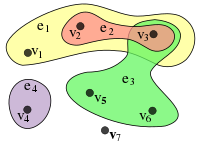HEBE（Large-Scale Embedding Learning in Heterogeneous Event Data）
对于只包含单种interaction的网络，一般都是在局部采集上下文(比如在文本中的，滑动窗口内的词视为上下文)，然后通过预测上下文来构建目标函数。  对于一个包含多种节点和边类型的网络，现有的方法PTE等，将所有object之间同时存在的interaction分解为几个分散的pairwise的interaction（比如论文网络，分解为论文-作者，论文-期刊/会议等），然后用传统的single-typed网络embedding方法求解。这种分解会丢失很多重要的信息，举个例子: A在期刊V上发表了论文

P1
$P_1$, B在期刊V上发表了论文

P2
$P_2$，但是A-B之间并没有合作关系（

A−P1−V−P2−B
$A-P_1-V-P_2-B$）。  HEBE 主要做的就是把跟一个事件相关的节点都关联到一个hyper edge中，以此来保留网络更多的信息。  如下图所示：  例1 DBLP数据只有一种event ：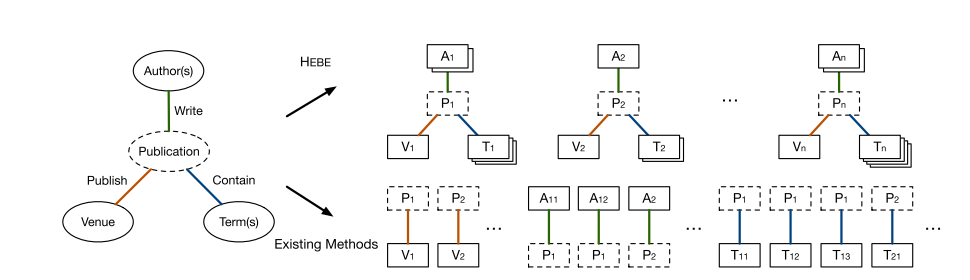例2 Yelp数据有两种event ：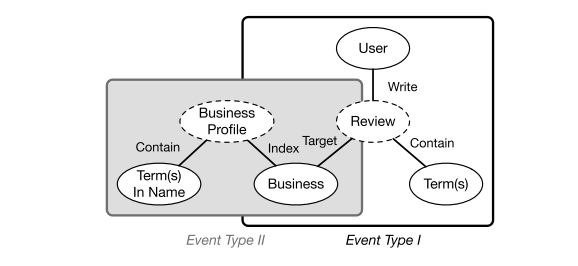几个基本定义
1. Information Network： 给定一个有T类objects的集合

X={Xt}Tt=1
$\mathcal{X} = \{X_t\}_{t=1}^T$ （ 其中

Xt
$X_t$是所有

tth
$t^{th}$类的object的集合），信息网络就是

G=(X,E)
$\mathcal{G}=(\mathcal{X}, \mathcal{E})$，

E
$\mathcal{E}$是连接两个object的边。如果

T≥2
$T \geq 2$， 那么是异质(heterogeneous)网络；如果T=1，那么是同质（homogeneous）网络。  2. 事件(event)：

Qi
$Q_i$可以表示为

<Vi,wi>
<script type="math/tex" id="MathJax-Element-14">

</script>，其中

wi
$w_i$ 是事件

Qi
$Q_i$的权重；

Vi={Vti}Tt=1
$V_i=\{V_i^t\}_{t=1}^T$，并且

Vti⊆Xt
$V_i^t \subseteq X_t$表示的是属于t类型的object的集合。

3. 超边：

Hi
$H_i$刻画事件Q_i\$，它把与事件的所有相关objects看作一个整体。

4. Subevent：子事件就是从每个object类型中均匀地采样出一个object组成地事件。现实的场景中，一个事件中的不同object类型对应的object数目

|Vti|≥1
$|V_i^t| \geq 1$（比如：一篇论文对应多个作者，多个term，却只对应一个venue）。对于一个事件

Qi={Vi,wi},Vi={a1,a2,a3}∪{t1,t2,t3}∪{v1}
$Q_i = \{V_i, w_i\}, V_i=\{a_1,a_2,a_3\}\cup \{t_1,t_2,t_3\} \cup \{v_1\}$。那么我们以1/（2*3）的概率可以得到子样本

Qi,s=a2,t2,v1
$Q_{i,s}={a_2,t_2,v_1}$，相应的子样本的权重为

wi/(2×3)
$w_i/(2 \times3)$。这也就造成了，不同的event有相同的Subevent。

模型构建
事件(event)的相似性：在event相关的所有object中，选出一个作为target，然后用剩余的objects作为上下文C预测这个object。并且，作者将target的候选集，定义在与target同类型的几点上，比如target是u，

u∈X1
$u \in X_1$并且

u∉C
$u \notin C$，条件概率定义为：

P(u|C)=exp(S(u,C))∑v∈X1exp(S(v,C))

\begin{equation}
\mathbb{P}(u|C)= \frac{exp(S(u,C))}{\sum_{v \in X_1}exp(S(v,C))}
\end{equation}
S是一个scoring function， 表示u/v与上下文C的相似度。假设上下文是

C={c1,c2,⋯,cT}
$C=\{c_1,c_2, \cdots, c_T\}$，并且对象u的向量表示为

w⃗ u∈Rd
$\vec{w}_u \in \mathcal{R}^d$，那么S函数可以表示为：

S(u,C)=<w⃗ u,1T−1∑t=2Tw⃗ ct>

\begin{equation}
S(u,C)=<\vec{w}_u, \frac{1}{T-1}\sum_{t=2}^T\vec{w}_{c_t}>
\end{equation}

为了保留object之间的相似性，最小化

P(u|C)
$\mathbb{P}(u|C)$和经验分布

Pˆ(u|C)=
$\widehat{\mathbb{P}}(u|C) =$之间的KL距离（假设：目标object类型为t，对应的上下文为

Ct
$C_t$，

Pt
$\mathcal{P}_t$是

Ct
$C_t$的采样空间

Pt={Ci,t}Ni=1
$\mathcal{P}_t = \{C_{i,t}\}_{i=1}^N$）：

L=−∑Tt=1γt∑Ct∈PtλCtKL(Pˆ(⋅|C),P(⋅|C))

\begin{equation}
\mathcal{L}=-\sum\nolimits_{t=1}^T \gamma_t \sum\nolimits_{C_t \in \mathcal{P}_t }\lambda_{C_t} KL(\widehat{\mathbb{P}}(\cdot|C),\mathbb{P}(\cdot|C))
\end{equation}

其中\gamma_t 为t类型的重要性参数，本文设置为

γ1=γ2=⋯=γT
$\gamma_1 =\gamma_2= \cdots = \gamma_T$；

λCt
$\lambda_{C_t}$表示上下文

Ct
$C_t$的重要性：

λCt=∑Ni=1wiI{Ct=Vi∖ui,t}

\begin{equation}
\lambda_{C_t}=\sum\nolimits_{i=1}^N w_i I_{\{C_t = V_i\setminus u_{i,t} \}}
\end{equation}
其中，

Pi,t
$\mathcal{P}_{i,t}$是

Pt
$\mathcal{P}_t$在

Vi
$V_i$上的子集。

I{⋅}
$I_{\{\cdot\}}$是一个二元指示函数。因而，

λCt
$\lambda_{C_t}$可以直观的解释为：以

Ct
$C_t$作为自己的超边加权数量。

基于

λCt
$\lambda_{C_t}$上式可以化简为：

L=−∑Tt=1∑Ct∈PtλCtKL(Pˆ(⋅|C),P(⋅|C))=−∑Tt=1∑Ct∈Pt∑Ni=1wiI{Ct=Vi∖ui,t}⋅∑Nj=1Pˆ(uj,t|Ct)logPˆ(uj,t|Ct)P(uj,t|Ct)=−Cˆ+∑Tt=1∑Ni=1wilogP(ui,t|Vi∖ui,t)

\begin{equation}
\mathcal{L}=-\sum\nolimits_{t=1}^T \sum\nolimits_{C_t \in \mathcal{P}_t }\lambda_{C_t} KL(\widehat{\mathbb{P}}(\cdot|C),\mathbb{P}(\cdot|C)) \\
=- \sum\nolimits_{t=1}^T \sum\nolimits_{C_t \in \mathcal{P}_t} \sum\nolimits_{i=1}^N w_i I_{\{C_t = V_i\setminus u_{i,t} \}}
\\ \cdot \sum\nolimits _{j=1}^N \widehat{\mathbb{P}}(u_{j,t}|C_t) log \frac{\widehat{\mathbb{P}}(u_{j,t}|C_t)}{\mathbb{P}(u_{j,t}|C_t)}
\\ = -\widehat{\mathbb{C}} + \sum\nolimits_{t=1}^T\sum\nolimits_{i=1}^N w_i log \mathbb{P}(u_{i,t}| V_i \setminus u_{i,t})
\end{equation}

其中

Cˆ
$\widehat{\mathbb{C}}$是一个常数，经验分布的计算为：

Pˆ(ui,t|Ct)=wi∑Nj=1wjI{Ct=Vj∖uj,t}

\begin{equation}
\widehat{\mathbb{P}}(u_{i,t}|C_t) = \frac{w_i}{\sum\nolimits_{j=1}^N w_j I_{\{C_t = V_j \setminus u_{j,t}\}}}
\end{equation}

对于多事件（event）类型的网络，目标函数可以写为：

L=∑Kk=1Lk

\begin{equation}
\mathcal{L} = \sum\nolimits_{k=1}^K \mathcal{L}_k
\end{equation}

优化（Noise Pairwise Ranking）
直接优化目标函数

L
$\mathcal{L}$计算量比较大，每次计算条件概率

P(u|Ct)
$\mathcal{P}(u|C_t)$都要遍历多有的属于t类型的object。noise contrastive estimation (NCE)和 negative sampling（NEG）都是把这个问题作为分类问题来解决的。但是负采样的方法，受超参数

k
$k$（负样本的数量）的影响，为了规避这个问题，本文提出了Noise Pairewise Ranking（NPR）的方法。相对比而言，NCE和NEG是判别模型，NPR是生成模型。
通过化简，条件概率可以表示为：
P(u|C)=11+∑v≠uexp(S(v,C)−S(u,C))\begin{equation}
\mathcal{P}(u|C)= \frac{1}{1+ \sum_{v \neq u} exp(S(v,C) -S(u,C))}
\end{equation}  然而，作者并没有直接优化上式，而是用一小部分的噪音样本

X1∖u
$X_1 \setminus u$，单个的节点可以表示为：

vn
$v_n$。优化的概率是：

P(u>vn|C)=σ(−S(vn,C)+S(u,C))

\begin{equation}
\mathcal{P}(u >v_n|C)=\sigma(-S(v_n,C) + S(u,C))
\end{equation}
直观解释，给定上下文C，最大化观测到目标节点u而不是负样本

vn
$v_n$的概率。特别是，很容易验证

P(u|C)>∏vn≠uP(u>vn|C)
$\mathcal{P}(u|C) > \prod\nolimits_{v_n \neq u} \mathcal{P}(u>v_n|C)$

得到的节点对排序结果

P(u>vn|C)
$\mathcal{P}(u >v_n|C)$与Bayies Pairwise Ranking（BPR）是很相似的。但是，BPR的负样本来自于从隐士反馈，NPR是基于条件概率的soft max定义近似推到出来的，而且负样本来自于噪音分布。因而，条件概率近似为：

P(u|C)∝Evn∼P(n)logP(u>vn|C)

\begin{equation}
\mathcal{P}(u|C) \propto E_{v_n \thicksim P(n)} log \mathcal{P}(u>v_n|C)
\end{equation}
在实验中作者采用的是异步梯度下降的方法。为了避免实验结果陷于局部最优（在计算scoring函数时，每个类型的object做了平均，这就导致object数量少的类型对应的object权重大，反之则权重小），作者根据节点类型调整步长：

βt=αtη
$\beta^t = \alpha^t \eta$，

αt=|Xt|maxTt′=1{|Xt′|}
$\alpha^t =\frac{|X_t|}{max_{t^{'}=1}^{T}\{|X_{t^{'}}|\}}$是梯度系数。实验结果证明HEBE是适用于大规模网络的，实验数据中 DBLP有1,938,912篇论文。

参考：  HEBE：Large-scale embedding learning in heterogeneous event data  Embedding learning with events in heterogeneous information networks
展开全文embedding html5
• 网络游戏-基于异质网络时态语义路径相似度的人物唯一性识别方法.zip
• 文章目录写在前面前两章简单阅读异质网络的基本概念基于原路径的挖掘异质网络的学习表示浅层模型基于分解的方法基于元路径的随机游走的方法基于关系的方法深度模型基于自动编码器基于生成对抗网络基于强化学习基于图...


文章目录
写在前面前两章简单阅读异质网络的基本概念基于原路径的挖掘
异质网络的学习表示浅层模型基于分解的方法基于元路径的随机游走的方法基于关系的方法
深度模型基于自动编码器基于生成对抗网络基于强化学习基于图神经网络
浅层模型与深层模型对比与知识图谱表示学习的区别与联系
应用场景商业领域网络安全医学领域
未来发展方向

写在前面
该文是针对该文章的阅读，主要关注在后三章，对后三章进行理解与扩展，并读了部分后三章所引用文章，因为前两章都是简单的基本概念，所以这里不详述。（本文是将我平常的工作记录、阅读笔记直接拷贝过来）论文链接：异质信息网络分析与应用综述（石川）
前两章简单阅读

整篇文章关注于以下几点：
异质信息网络的概念异质信息网络原路径的数据挖掘方法异质信息网络的表示学习技术异质信息网络的实际应用 绪论介绍了异质网络为什么出现以及异质网络出现的意义

异质网络的基本概念

信息网络；balaba异质/同质网络：节点与边的类型是否多于1网络模式：就像是知识图谱的本题，是构建异质网络所遵循的一种基本概念，或是基本规则，网络模式是类，而实际异质网络算是类的一种实现方式下面是从语义上去探索元路径：从一个类型到达另一个类型，中间会经历不少的其他类型，也会经历不少的其他边，这些是一条元路径P。而从一个具体的对象到达另外一个对象，就是该元路径P的一个实例路径p。
元路径本质上抽取了异质网络的子结构其体现了路径上的丰富语义信息异质网络分析的基本语义捕捉方法其缺陷也很明显，所以应运而生升级的语义捕捉方法们 受限元路径：施加约束的元路径，针对特定约束的元路径，更加具体，针对
可以约束类型的属性值或者说，不是约束，而是展示 加权元路径：展示关系属性值的元路径，可以说是对关系的属性值进行刻画
展示关系的强弱，比元路径具有更深刻的语义 元结构/元图：
融合了多条原路径蕴含着更多的语义信息 后面的异质网络举例就没什么好说的了，不过那些数据集我倒是觉得有点意思后面还提到了知识图谱和异质网络，awsl，舒服了

基于原路径的挖掘

相似性度量推荐（大致上看了一下，是基于元路径）分类聚类及其他元路径选择：这里不细看，

异质网络的学习表示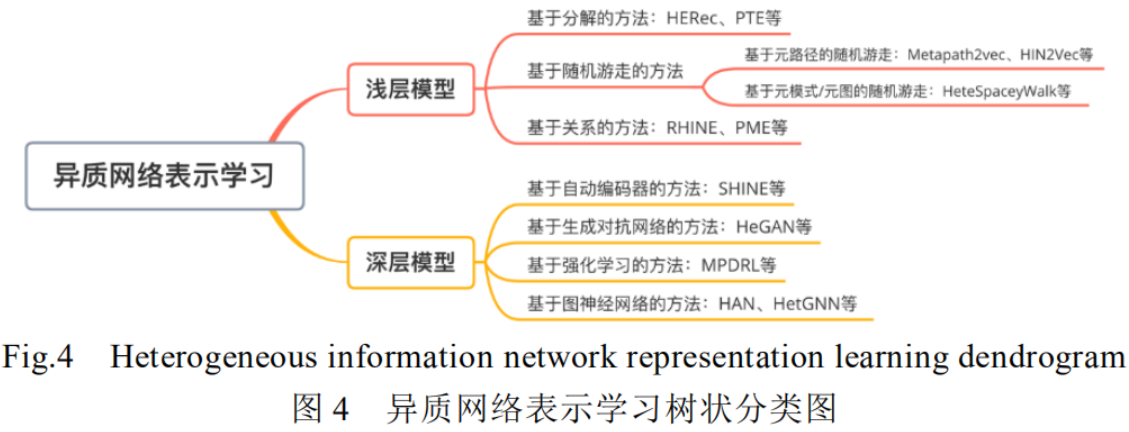浅层模型
基于分解的方法

概念为分治思想，将大型复杂的网络分解成简单的小网络（同质），分别进行表示学习，然后进行信息融合HERec：论文因为是做推荐的，所以它只关注user和item，因此，在论文中只选择了那些user类型或item类型作为起点的元路径，然后进行随机游走，从而生成多个元路径所对应的节点序列，此时，其将其中非起点类型的节点全部删去，从而形成了一个同质的元路径。从而将整个异质网络分解成了多个同质网络。其中改论文获得所有节点序列的方式是随机游走，所以这是一个基于元路径的随机游走
针对每个节点，便可以获得一些元路径的随机游走的表示，将这些可以融合成一个向量来表示该节点它是给定异质网络，给定元路径，然后根据随机游走生成该元路径的一些实例，之后再进行删点这里的随机游走在于，给定元路径，根据元路径的规则（类别）随机选择实例对象这里主要是从异质网络分解成同质网络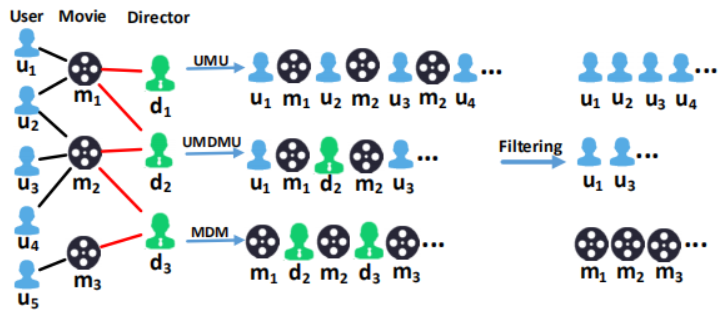尹赢 结合不同元路径将异质信息网络转换成带权同质子图
大致的流程是：利用先验知识，根据同类节点间不同元路径的语义信息对不同元路径设置不同权重，然后根据权重及路径数构建出代权重的同质子图，通过同质子图上进行带偏置的随机游走得到同类节点序列（偏置意为有方向引导）。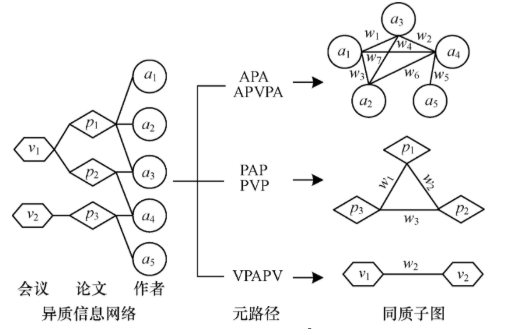这个图救命了啊，懂了，根据不同元路径对应的不同权值，来将整个网络给变成不同的同质子图 PTE和EOE都是对异质网络进行简单的拆分上述这些分解会损失异质邻居的信息，只关注到了同质的邻居信息，这个···也是对我之前提的方法的否定，大哭

基于元路径的随机游走的方法

随机游走本身不受控制但是基于元路径的话，就相当于是在随机游走时有了约束大概理解了node2vec的作用以及机理：大概是，我对一个点，可以设定随机游走的路径个数，然后抽取这个个数的随机游走路径，这些路径都包含该节点，然后该节点就可以用这些路径来表示。所以表示该节点的向量，就包含了这个节点以及其周围的结构信息而基于元路径的随机游走也基本上是这样的，只不过是受约束的，受元路径的约束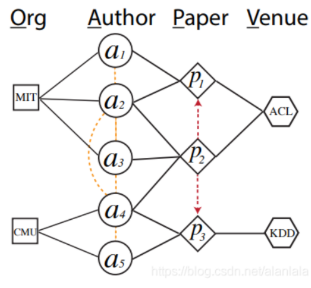eg：OAPVPAO

基于关系的方法

RHINE模型，是将关系分为两类，一类是一对多，一类是一对一，分别对两种关系类别分开建模。BHIN2vec：
带偏置的随机游走因为不同关系的数量不同，所以损失不一样，所以在进行随机游走的的时候加上误差，尽力使得平衡 后面的不看了这里关于关系系的有点懵，不是太理解

深度模型
慢慢的看博客，论文翻译，要好多了，生成对抗网络理解的多的多了
基于自动编码器

利用神经网络构建编码其学习节点属性表示的同时保持网络结构特性对不同元路径的信息进行编码，之后综合信息进行联合编码关于这一块，更多的抽象理解成GAN中的生成器

基于生成对抗网络

利用生成对抗网络来进行表示生成对抗网络的强大之处在于可以自动学习原始真实样本集的数据分布则其就可以用来学习并生成逼真的样本生成器与判别器进行对抗博弈来捕获语义信息进行关系感知在HeGAN中，判别器和生成器都被设计成关系感知的，对于任何关系，判别器都可以分辨出一个节点的真假而生成器可以模仿真节点对来生成假节点对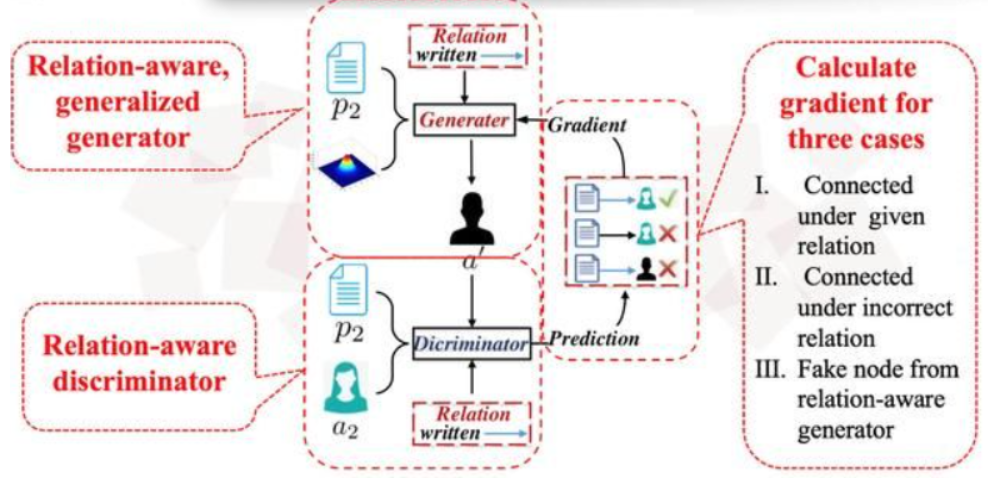基于强化学习

强化深度学习是强化学习+神经网络其主要关键点在于强化学习，而不是神经网络，神经网络只是让强化学习能处理复杂的高维信息强化学习主要在于根据环境来决定如何行动边获得样例边更新模型，然后根据当前模型来进行指导下一步行动而下一步行动是考虑环境，观察环境，并考虑基于当前环境，不同行为所能够带来的reward是不一样的基于此来进行选择此处是针对元路径的选择困境，将下有任务性能作为rewardstate是已选择的链接类型顺序action来选择用于学习或终止训练的特定类型链接在异构星型网络的节点表示学习问题上，不同类型边的训练顺序会影响模型的表现效果所以在涉及到这个学习的过程中，用深度强化学习来进行确定边的训练顺序

基于图神经网络

这一块的大概意思是将邻域信息聚合做为消息传递给邻居节点（用周围信息来描述该节点）。也就是之前理解的那种进行随机游走，将节点周围的结构信息给也刻画到该节点上（只能说是有点像）进行修改，例如基于分层注意力机制学习每个节点的个性化元路径权重而对于异质网来说，如何设计合适的聚合函数来捕获领域包含的的语义是关键

浅层模型与深层模型对比

浅层模型主要关注在异质网络的结构，很少利用属性等额外信息浅层模型不易描述额外信息与结构信息的关系，同时建模两者困难深层模型的学习能力支持复杂的的建模方式，能够整合网络的结构和属性信息浅层模型缺乏非线性表示能够力，但高效且易于并行（自然，整合的东西少，本身神经网络就要慢一些）深层模型表示能力强，但是容易拟合噪音、时空复杂度高且调参繁琐

与知识图谱表示学习的区别与联系

知识图谱的表示学习以及问题知识图谱以图的形式表现客观世界中的尝试和事实可以给人工智能系统提供可处理的先验知识知识图谱表示学习将知识图谱中的实体和关系 学习为 包含语义信息的低维向量表示传统异质网络表示学习关注 拓扑结构知识图谱节点丰富，连接类型丰富，难以直接引用元路径等传统异质网络挖掘方法目前主流的表示学习方法Trans系列

知识图谱是无模式的异质网络，本质上属于异质网络范畴为了平衡效率和效果，知识图谱表示学习方法忽略网络本身复杂结构（如何将简单模式的异质网络迁移至复杂模式的知识图谱）知识图谱有本题数据模型，传统异质网络表示如何将基于本体和规则的推理方法在语义层刻画

应用场景

之前的是基础数据挖掘人物除此之外 在商业、安全、医学等领域有许多实际的场景

商业领域

异质网络建模可以涵盖多类型节点及其之间的交互，整合丰富甚至异构的信息源，从而全面地刻画节点特征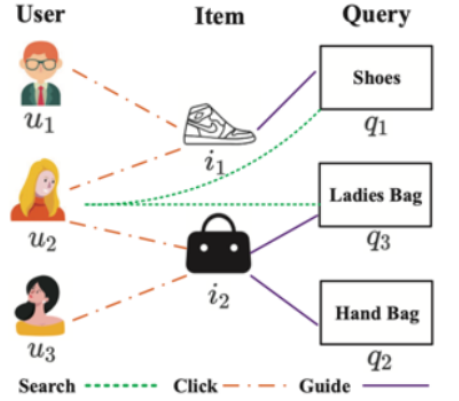最广泛的应用：推荐传统的推荐：仅考虑用户与物品的交互实际上的推荐应该：用户、商品、店铺、朋友之间的交互MEIRec：建模异质网络，提出基于元路径的异质图神经网络学习意图推荐中的用户表示Yu建模基于位置的社交网络，用贪心算法进行兴趣点 组推荐HIE通过建模文字与响应模式等信息，预测用户的个性特征CIKM最佳应用论文将咸鱼app中的用户、商品和评论建模为二分网络，并基于图神经网络进行垃圾评论过滤，减少其对用户选择的影响另一个应用：套现用户检测检测具有极高贷款违约率的用户常规方法基于统计特征训练分类器、HACUD利用属性异质网络建模蚂蚁金服信用支付中用户与商家间的交互，并基于原路径和层次注意力机制学习用户表示另一个应用：在线租车平台希望提升用户乘车体验并预测用户行程PHINE建模驾驶员、乘车和位置等信息TDP利用行程起止点来预测用户短期出行可能

网络安全

恶意软件检测：传统方法基于签名识别KDD最佳论文，提出HinDroid将安卓应用程序、相关API及其丰富关系建模成异质网络，基于不同元路径度量安卓应用的相似性。最终利用多核学习加权相似性来进行预测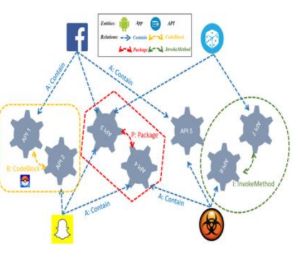AiDroid分类学习节点表示，利用深度神经网络进行监测aCyber提出对抗攻击模型和防御模型来提升监测的鲁棒性

恶意账户检测Liu从支付宝异质子图中自适应学习嵌入表示，利用注意力机制区分不同类型节点的重要性OSNE将犯罪和恐怖主义活动建模成异质网络，来识别恐怖分子iDetector建模地下论坛，基于不同原结构表征帖子间的相关性并进行有效融合uStyle-uIDy建模文本和照片，提出基于书写及摄影风格识别毒品贩子

异常事件检测一个常评论动作电影的用户突然评论了情感类电影就是异常Fan提出同时包含实体属性和二阶结构深度异质网络嵌入方法Ranjbar等人提出基于张量分解和聚类的异常检测方法

医学领域

疾病诊断电子健康记录（EHR）有患者临床实践的详细记录，结构、语义丰富HeteroMed使用异质网络对临床数据进行建模，利用元路径捕获有助于疾病诊断的重要语义HinOPU框架利用异质网络建模推特中用户和推文间的关系，基于元图表征用户间的语义相关性进行阿片类药物使用者的预测

基因分析以往的方法只关注基因本身，忽视了相关的生物网络拓扑结构中的信息PLPIHS建模lncRNA-蛋白质网络，基于HeteSim（一种相关性计算方法，基于元路径）计算lncRNA-蛋白质对的相关性评分来推断其相互作用马毅基于HeteSim尖酸治病基因间的相关性

未来发展方向

面向多模态数据的异质网络构建与分析方法现有工作致力于关系数据库类的结构化数据建模文本、图像和多媒体？存在不少难题：
利用半结构化的异质网络建模多模态数据时，节点和边关系不明确且复杂（如何从多模态数据中提取出合适的对象和关系）异质网络表示学习和多模态数据表示学习 如何有机融合

面向复杂网络数据的异质网络分析方法实际复杂网络的特点变成了难题：实际网络动态异质：不断新节点产生，新交互实际网络规模巨大：算法的时间复杂度，响应时间实际网络模式丰富：知识图谱？难以描述，实际网络的连接包含丰富信息：社交异质网络中的关注、转发等是有向的，···

面对深度计算的异质网络表示学习异质图神经网络的内部机制：聚合邻居信息异质图神经网络的鲁棒性：（研究表明）其易受对抗攻击异质网络表示学习的可解释性：eg，商品推荐依据元路径的注意力权重的推荐理由？异质网络与知识的融合：如何融合知识与异质网络产生足够的泛化能力

更多的实际应用：应用不少，上面讲到已经有实际应用，商业，医学，网络安全但是其他领域引用的还是太少，尚待发掘

展开全文神经网络 知识图谱 异质信息网络
• 科研社交网络中基于异质网络分析的列表级排序学习推荐方法研究.pdf
• 网络游戏-异质网络间进行中通讯期间的转移的多重模式移动站台.zip
• 行业分类-物理装置-一种基于异质网络表示学习的链路预测方法.zip
• 为了分析DBLP数据中的多种类型的实体信息，挖掘其中特定的知识，首先根据异质网络Graph OLAP（图联机分析处理）模型，建立相应的数据仓库模型；然后依据实体维的概念，构建多维异质图立方模型；最后针对 Graph OLAP...图挖掘 数据仓库
• 人工智能AI源码解析-基于对抗解耦器的异质网络嵌入
• 由多种类型的实体和关系构成的异质信息网络逐渐成为社会网络分析的研究热点。作为异质信息网络的一个独特属性，元路径包含了丰富的语义信息。实际生活中的许多网络都包含带权值的链接，这使得不考虑链接上权值的传统...元路径 相关性搜索
• [Structural Deep Embedding for Hyper-Networks](https://arxiv.org/abs/1711.10146) 是在hyperedge(超边是不可分解的)的基础上保留object的一阶和二阶相似性，学习异质网络表示。于与HEBE的区别在于，本文考虑了...hypernet embedding similarity
•研究论文
• 从进化和异质网络中表征和预测社区成员 摘要 近年来，从web数据中挖掘不同类型的社区吸引了大量的研究工作。然而，现有的社区挖掘技术都没有考虑到web数据的动态性和异构性。在本文中，我们提出了从异构web数据的...
• 异质信息网络研究现状及未来发展 一、引言 现实生活中的大多数实际系统是由大量相互作用、类型不同的组件构成，当前的分析方法通常将其建模为同质信息网络（Homogeneous information network）。采用同质网络的建模...数据挖掘 算法 深度学习
• metapath2vec: Scalable Representation Learning for Heterogeneous Networksmetapath2vec https://dl.acm.org/citation.cfm?id=3098036是17年发表的，使用基于meta－path的随机游走重构节点的...，并用异质的skip...meta-path Embedding Skip-gram
• ## 异质信息网络和知识图谱

千次阅读 多人点赞 2020-10-31 11:36:00
最近在研究异质信息网络，发现知识图谱无论是在建模方式还是下游任务都与异质信息网络有很大的相似性。 在这里简单介绍一下知识图谱的相关概念和从网上找来的知识图谱嵌入综述类的文章或者博客进行总结。 比较分析...数据挖掘 机器学习 深度学习
• wincc通过OPC跨网络访问异质网络上的PLC文件...